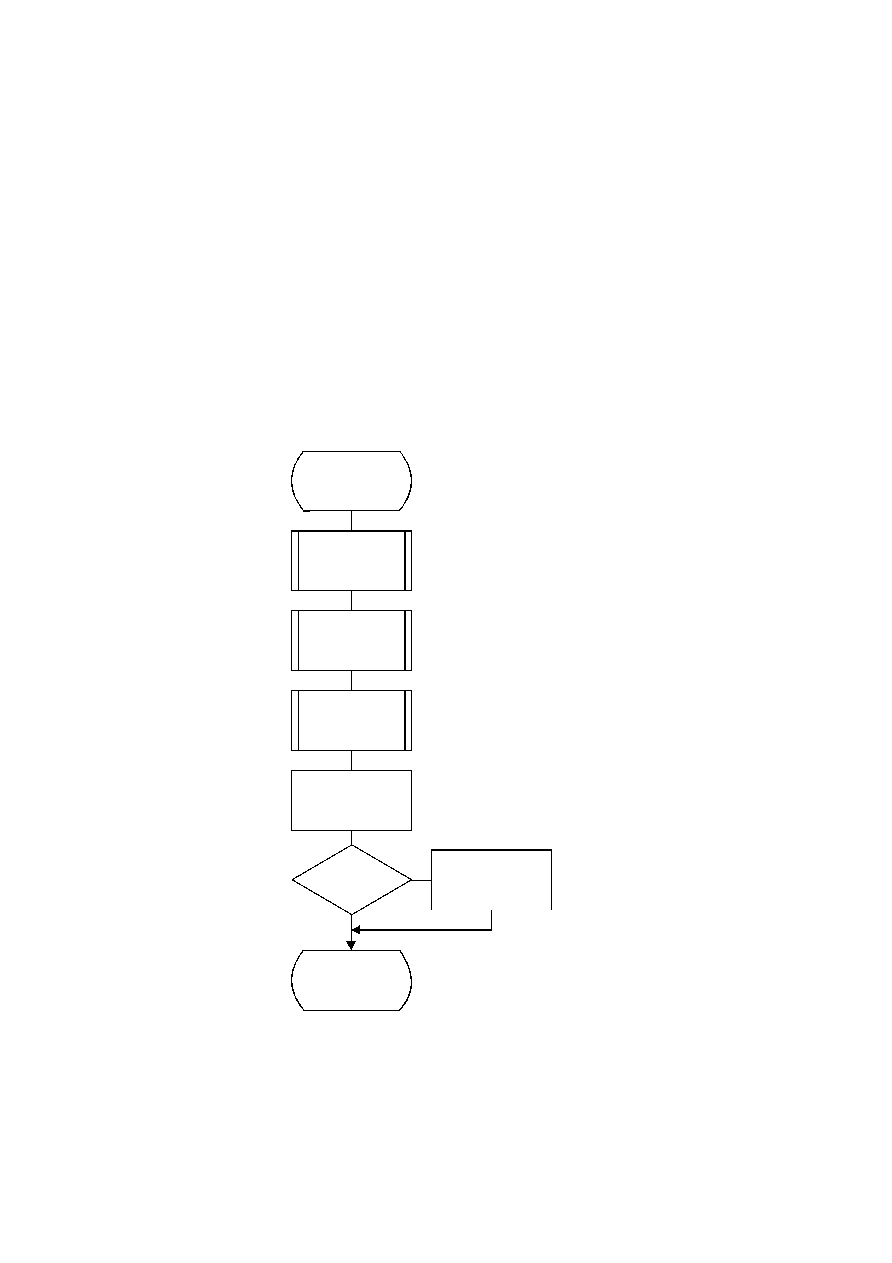ISO/IEC 10918-1 : 1993(E)
In Figure F.20, K is the index to the zig-zag sequence position. For the sequential scan, Kmin
=
1 and Se
=
63. The
decision at the top of the loop is the EOB decision. If the EOB occurs (D
=
1), the remaining coefficients in the block are
set to zero. The inner loop just below the EOB decoding decodes runs of zero coefficients. Whenever the coefficient is
non-zero, "Decode_V" decodes the sign and magnitude of the coefficient. After each non-zero coefficient is decoded, the
EOB decision is again decoded unless K
=
Se.
F.2.4.3
Decoding the binary decision sequence for non-zero DC differences and AC coefficients
Both the DC difference and the AC coefficients are represented as signed two's complement 16-bit integer values. The
decoding decision tree for these signed integer values is the same for both the DC and AC coding models. Note, however,
that the statistical models are not the same.
F.2.4.3.1
Arithmetic decoding of non-zero values
Denoting either DC differences or AC coefficients as V, the non-zero signed integer value of V is decoded by the
sequence shown in Figure F.21. This sequence first decodes the sign of V. It then decodes the magnitude category of V
(Decode_log2_Sz), and then decodes the low order magnitude bits (Decode_Sz_bits). Note that the value decoded for Sz
must be incremented by 1 to get the actual coefficient magnitude.
TISO1530-93/d091
Decode_V(S)
Decode_sign_of_V
Decode_log2_Sz
Decode_Sz_bits
V = Sz + 1
SIGN = 1
?
Yes
V = ­V
Done
Figure F.21 ­ Sequence of procedures in decoding non-zero values of V
No
Figure F.21 [D91] = 15.5 cm = 606 %
114
CCITT Rec. T.81 (1992 E)